CATEGORIES:

# Lexicographic order

The words in a dictionary are listed in alphabetic, or lexicographic, order, which is based on the ordering of the letters in the alphabet. This is a special case of an ordering of strings on a set constructed from a partial ordering on the set.

The lexicographic ordering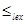on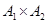is defined by specifying that one pair is less than a second pair if the first entry of the first pair isles than (in A1) the first entry of the second pair, or if the first entries are equal, but the second entry of this pair is less than (in A2) the second entry of the second pair. In other words,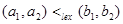, either if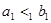or if both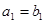and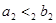. We obtain a partial orderingby adding equality to the ordering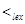on.

Example. Determine whether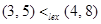, whether, whetherin the poset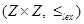, whereis the lexicographic ordering constructed from the usual ≤ relation on Z.

A lexicographic ordering can be defined on the Cartesian product of n posets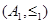,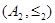, …,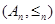. Define the partial orderingon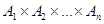byif, or if there is an integer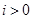such that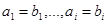and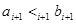.

We can now define lexicographic ordering of strings. Consider the strings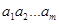and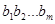on a partially ordered set S. Suppose these strings are not equal. Let t be the minimum of m and n. The definition of lexicographic ordering is that the stringis less thaniffor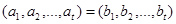and m < n wherein this inequality represents the lexicographic ordering of S t.

Example. Consider the set of strings of lowercase English letters. Using the ordering of letters in the alphabet, a lexicographic ordering on the set of strings can be constructed. A string is less than a second string if the letter in the first string in the first position where the strings differ comes before the letter in the second string in this position, or if the first string and the second string agree in all positions, but the second string has more letters. This ordering is the same as that used in dictionaries. For example, discreetdiscrete, since these strings differ first in the seventh position, and et. Also, discreetdiscreetness, since the first eight letters agree, but the second string is longer. Furthermore, discretediscretion, since discretediscreti.

Date: 2015-01-02; view: 561

 <== previous page | next page ==> Partial orderings | Hasse diagrams
doclecture.net - lectures - 2014-2020 year. Copyright infringement or personal data (0.003 sec.)